# Accelerated Depreciation

A depreciation method in which an asset loses book value at a faster (accelerated) rate than traditional depreciation methods

## What is Accelerated Depreciation?

Accelerated depreciation is a depreciation method in which an asset loses book value at a faster (accelerated) rate than it would using traditional depreciation methods such as the straight-line method. Therefore, under accelerated depreciation, an asset faces greater deductions in its value in the earlier years than in the later years. Accelerated depreciation is often used as a tax-reduction strategy.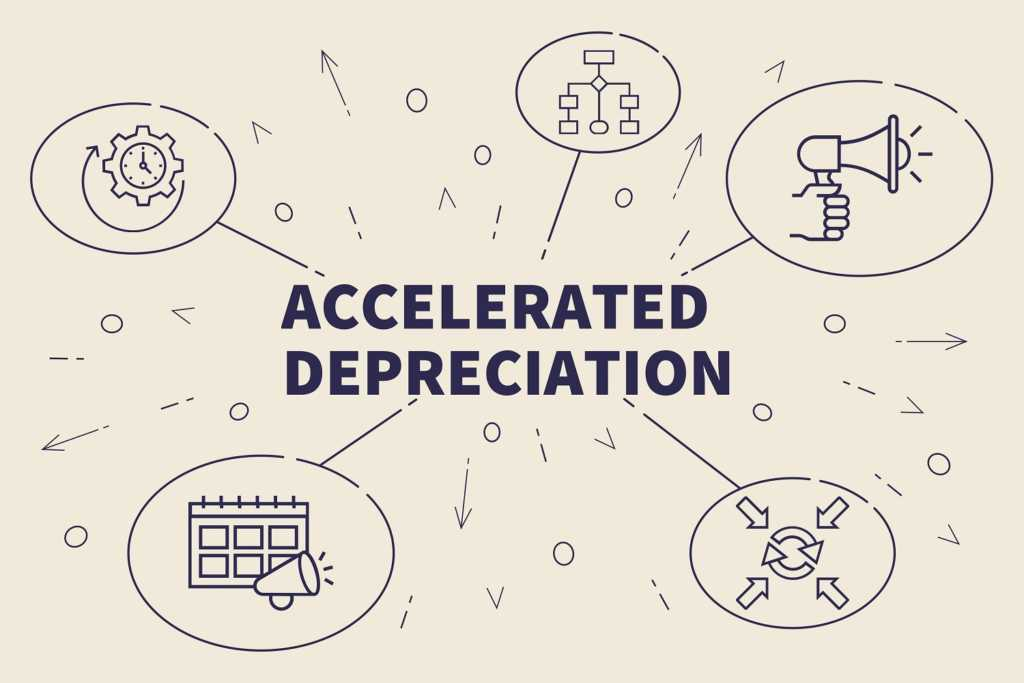### Popular Accelerated Depreciation Methods

The most popular accelerated depreciation methods are the double declining balance method and the sum of the years’ digits method. The formula for calculating depreciation using each of these methods is given below:

#### 1. Double declining balance method:

Double declining balance = 2 x Straight-line depreciation rate x Book value at the beginning of the year

#### 2. Sum of the years’ digits method:

Applicable percentage (%) = Number of years of estimated life remaining at the beginning of the year / SYD

Where:

SYD = n(n+1) / 2

• SYD stands for sum of the years’ digit
• n = number of years

### Example of the Double Declining Balance Method

CFI Company purchases a machine for \$100,000, with an estimated salvage value of \$10,000 and a useful life of 5 years. The straight-line depreciation rate is 20%.

The double declining balance depreciation method calculation is: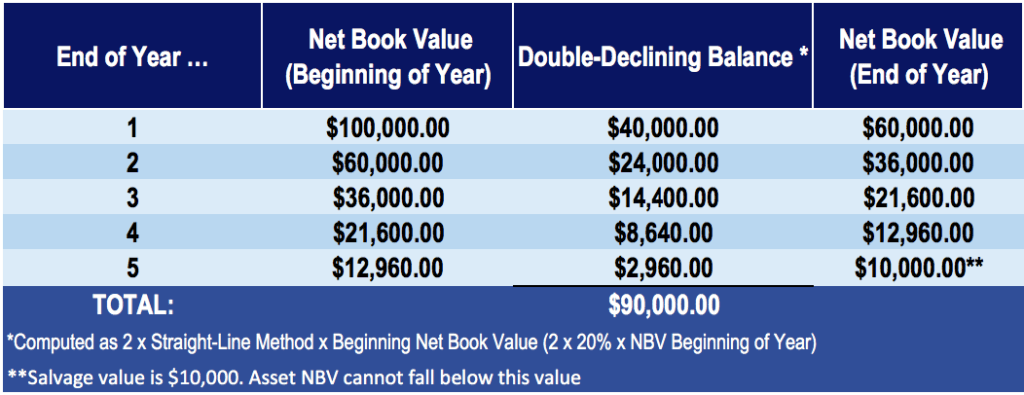### Example of the Sum of the Years’ Digits Method

CFI Company purchases a machine for \$100,000 with an estimated salvage value of \$10,000 and a useful life of 5 years. The straight-line depreciation rate is 20%.

The sum of the years’ digits method calculation is: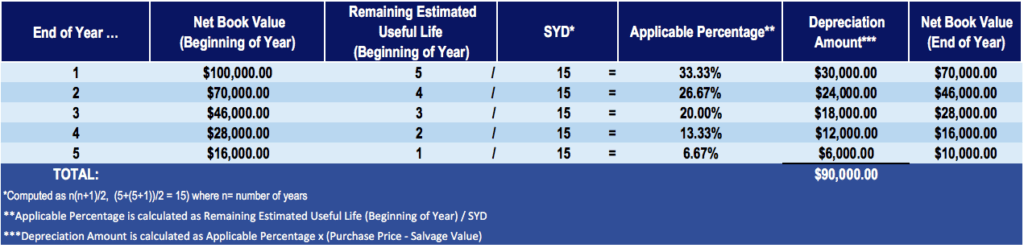### Comparing the Accelerated Depreciation Methods with the Traditional Straight-Line Method

Let us calculate the straight-line depreciation for the same example – a machine worth \$100,000, with an estimated salvage value of \$10,000 and a useful life of 5 years – and compare it to the accelerated methods of depreciation.

A table with the depreciation amounts each year for each method: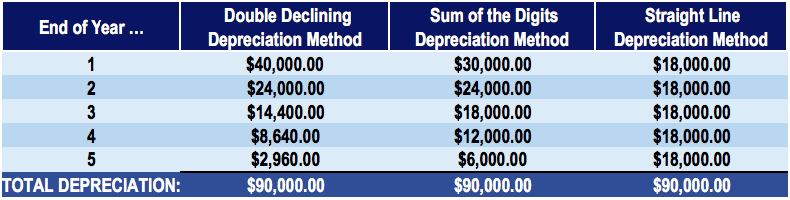A table with the end of year book values for each method: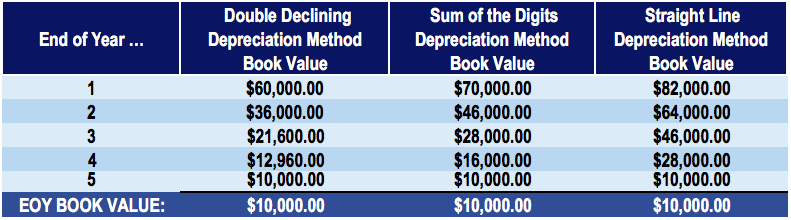Under all three methods, the total depreciation and book value at the end of the machine’s useful life is the same – \$90,000 in total depreciation and \$10,000 in ending book, or salvage, value.

### Financial Statement Impact of Different Depreciation Methods

Note from the tables above that the amount of depreciation in each year is different under varying methods. With the accelerated methods of depreciation (double declining and sum of the years’ digits), there is greater depreciation in the earlier years, as compared to the straight-line depreciation method. So, how do the accelerated methods of depreciation affect an asset’s value and the company’s net income?

The amount of depreciation of an asset affects the reported profits of a company (through the income statement). Therefore, the accelerated methods of depreciation skew the profits of the company and reveal lower profit in the earlier years of the asset’s acquisition. As the asset comes closer to the end of its useful life, it faces less annual depreciation, with the net effect of the company realizing a higher reported profit in those later years.

For example, consider a company that generates yearly revenues of \$100,000. For simplicity, assume that the only operating expense of the company is depreciation expense (no rent expense, wage expense, etc.). Notice the difference in operating income under an accelerated method of depreciation compared to a straight-line depreciation method: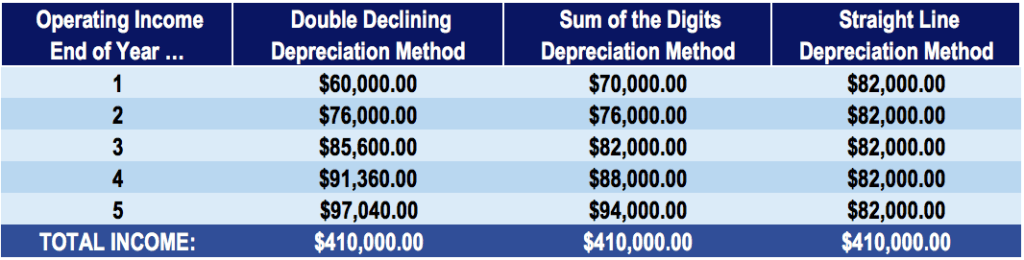As illustrated in the table above, an accelerated depreciation method results in lower reported profit in earlier years but higher profit in later years as compared to a traditional straight-line depreciation method.

### Tax Savings and Net Present Value

Companies often use rapid depreciation methods to reduce taxes in the early years of an asset’s life. It’s important to note that total tax deductions over the life of an assset will be the same no matter what method is used.  The only benefit of an accelerated method is the timing of the deductions. Rapid methods have more tax savings in early years and fewer savings in later years. Since managers of businesses take the Time Value of Money into consideration, it’s better to have the savings early, rather than later. This helps to improve the Net Present Value of the business.

Thank you for reading this article on accelerated depreciation methods and reasons why accountants and managers use them. CFI is the official provider of the Financial Modeling & Valuation Analyst (FMVA)™ certification program, designed to help anyone become a world-class financial analyst. To keep advancing your career, the additional CFI resources below may be useful:

• Accumulated Depreciation
• Depreciation Expense
• Depreciation Schedule
• Depreciation Methods Template